# 主成分分析(Principal component analysis, PCA)例子–Python

• 2
• 7,468 views
• A+

## 实验介绍

### 数据集介绍

The Iris Dataset contains four features (length and width of sepals and petals) of 50 samples of three species of Iris (Iris setosa, Iris virginica and Iris versicolor). These measures were used to create a linear discriminant model to classify the species. The dataset is often used in data mining, classification and clustering examples and to test algorithms.

Information about the original paper and usages of the dataset can be found in the UCI Machine Learning Repository -- Iris Data Set.

Just for reference, here are pictures of the three flowers species: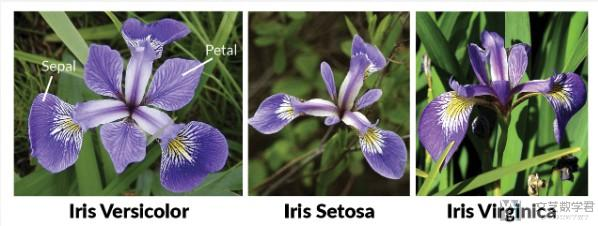### 实验内容介绍

1. import pandas as pd
2. import numpy as np
3. import matplotlib.pyplot as plt
4. from sklearn.decomposition import PCA
5. from sklearn.preprocessing import StandardScaler
6. %matplotlib inline

#### 导入数据集

1. df = pd.read_csv('./iris.csv', names=['sepal length','sepal width','petal length','petal width','target'])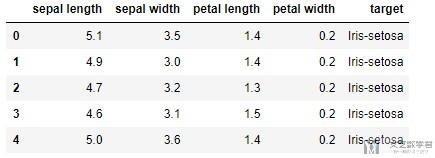#### 数据标准化

PCA is effected by scale so you need to scale the features in your data before applying PCA.(PCA会被数据的大小所影响, 所以在做之前, 我们需要先对数据进行标准化). 我们使用StandardScaler进行标准化, 标准化之后变为均值为0, 方差为1的数据.

1. features = ['sepal length', 'sepal width', 'petal length', 'petal width']
2. # Separating out the features
3. x = df.loc[:, features].values
4. # Separating out the target
5. y = df.loc[:,['target']].values
6. # Standardizing the features
7. x = StandardScaler().fit_transform(x)

1. # 查看标准化之后的数据
2. pd.DataFrame(data = x, columns = features).head()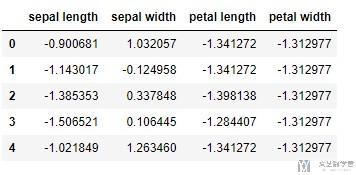#### 选择降维后的维数

1. pca = PCA(n_components=4)
2. principalComponents = pca.fit_transform(x)
3. pca.explained_variance_ratio_
4. """
5. array([0.72770452, 0.23030523, 0.03683832, 0.00515193])
6. """

1. # 进行可视化
2. importance = pca.explained_variance_ratio_
3. plt.scatter(range(1,5),importance)
4. plt.plot(range(1,5),importance)
5. plt.title('Scree Plot')
6. plt.xlabel('Factors')
7. plt.ylabel('Eigenvalue')
8. plt.grid()
9. plt.show()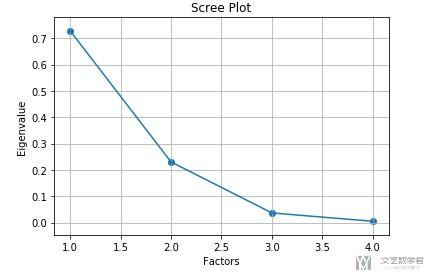#### 使用PCA降维

1. # 进行降维
2. pca = PCA(n_components=2)
3. principalComponents = pca.fit_transform(x)
4. # 查看降维后的数据
5. principalDf = pd.DataFrame(data=principalComponents, columns=['principal component 1', 'principal component 2'])
6. finalDf = pd.concat([principalDf, df[['target']]], axis = 1)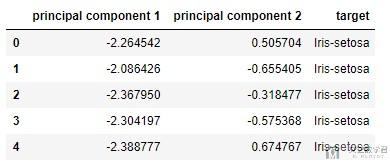#### 查看转换系数

1. pca.components_
2. """
3. array([[ 0.52237162, -0.26335492,  0.58125401,  0.56561105],
4.        [ 0.37231836,  0.92555649,  0.02109478,  0.06541577]])
5. """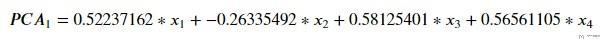1. (np.dot(x,pca.components_), np.dot(x,pca.components_))
2. """
3. (-2.2645417283949003, 0.5057039027737843)
4. """

1. # 对系数进行可视化
2. import seaborn as sns
3. df_cm = pd.DataFrame(np.abs(pca.components_), columns=df.columns[:-1])
4. plt.figure(figsize = (12,6))
5. ax = sns.heatmap(df_cm, annot=True, cmap="BuPu")
6. # 设置y轴的字体的大小
7. ax.yaxis.set_tick_params(labelsize=15)
8. ax.xaxis.set_tick_params(labelsize=15)
9. plt.title('PCA', fontsize='xx-large')
10. # Set y-axis label
11. plt.savefig('factorAnalysis.png', dpi=200)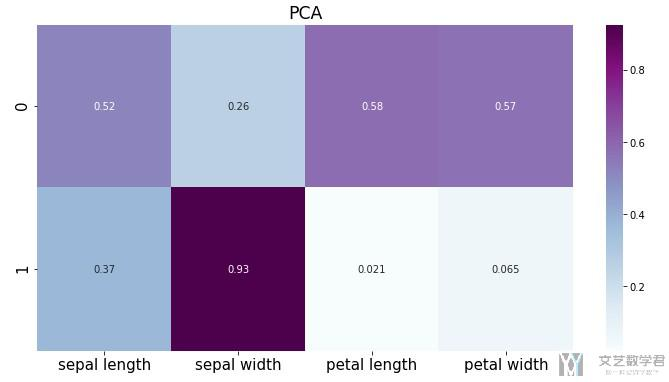#### 数据降维可视化

1. fig = plt.figure(figsize = (8,8))
3. ax.set_xlabel('Principal Component 1', fontsize = 15)
4. ax.set_ylabel('Principal Component 2', fontsize = 15)
5. ax.set_title('2 Component PCA', fontsize = 20)
6. targets = ['Iris-setosa', 'Iris-versicolor', 'Iris-virginica']
7. colors = ['r', 'g', 'b']
8. for target, color in zip(targets,colors):
9.     indicesToKeep = finalDf['target'] == target
10.     # 选择某个label下的数据进行绘制
11.     ax.scatter(finalDf.loc[indicesToKeep, 'principal component 1']
12.                , finalDf.loc[indicesToKeep, 'principal component 2']
13.                , c = color
14.                , s = 50)
15. ax.legend(targets)
16. ax.grid()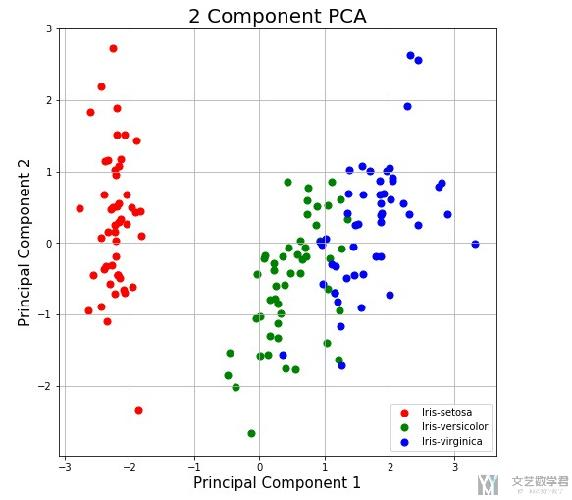#### 与因子分析(FA)比较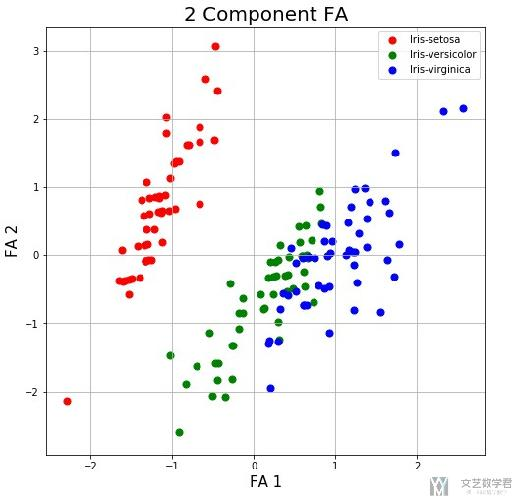## 项目代码

Github仓库Notebook: PCA(主成分分析)+FA(因子分析)

• 微信公众号
• 关注微信公众号
•• QQ群
• 我们的QQ群号
•## 目前评论：2   其中：访客  0   博主  0

•子夜

这个for循环是什么意思呢？
我尝试了你的方法，没有报错，但数据没有在图上显示出来，我想可能是这个for循环我没理解。

1. for target, color in zip(targets,colors):
2.     indicesToKeep = finalDf[‘target’] == target
3.     # 选择某个label下的数据进行绘制
4.     ax.scatter(finalDf.loc[indicesToKeep, ‘principal component 1’]
5.                , finalDf.loc[indicesToKeep, ‘principal component 2’]
•王 茂南 Admin

@子夜 这是对每一类的样本绘制不同的颜色. 前面定义了targets和color, 我们找出对应的target的index, 接着设置不同的颜色. 这里的for循环就是对不同的种类分别进行绘制不同的颜色.